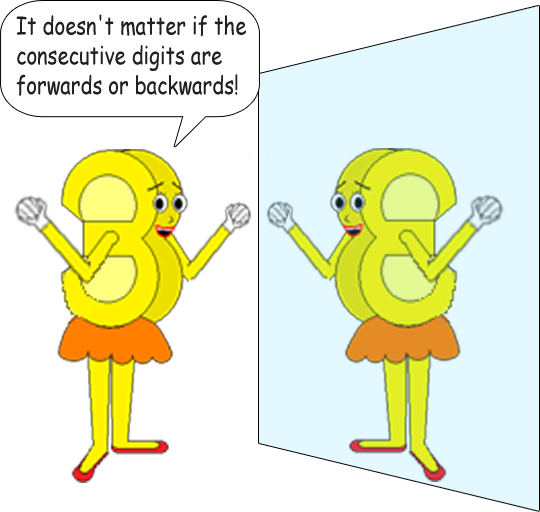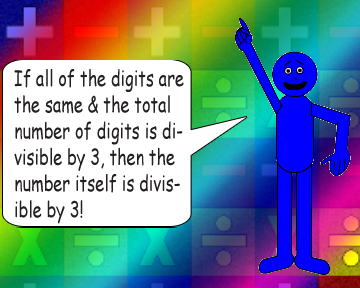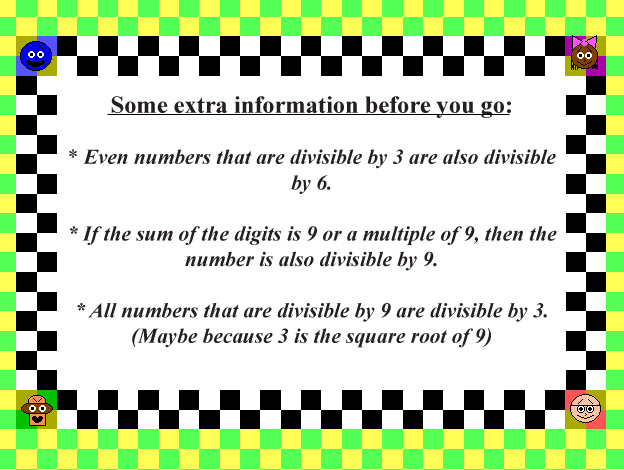# Dividing Numbers With Consecutive Digits

## Most numbers with consecutive digits are divisible by 3! Observe below:

012 ÷ 3 = 4

123 ÷ 3 = 41

234 ÷ 3 = 78

345 ÷ 3 = 115

456 ÷ 3 = 152

567 ÷ 3 = 189

678 ÷ 3 = 226

789 ÷ 3 = 263

[The pattern stops here since 9 is the greatest digit & 890 is NOT divisible by 3]

The number is especially divisible by 3 if the total number of digits is divisible by 3! (Have you noticed the padding zero I put on the number 12?) All the quotients in the example above have an integral difference of 37.

## But there's more you can do in this trick! You can skip digits so that only odd or even digits are used, or you can just use 0, 3, 6 & 9 - the single digit multiples of 3. (Yes, zero counts as a multiple, too.)

246 ÷ 3 = 82

468 ÷ 3 = 156

369 ÷ 3 = 123

135 ÷ 3 = 45

123,456 ÷ 3 = 41,152

123,456,789 ÷ 3 = 41,152,263

9,630 ÷ 3 = 3,210

147,741 ÷ 3 = 49,247

If the number of digits isn't divisible by 3 or the digits aren't consecutive, then the whole number isn't divisible by 3.

## 572 ÷ 3 = 190 + 2/3 (Not an integer!)

There's this special rule for divisibility by 3:

If the sum of all the digits in a number is divisible by 3, then the number itself is also divisible by 3.

## This often happens if the number has consecutive digits or the total number of digits is divisible by 3!987 ÷ 3 = 329

876 ÷ 3 = 292

765 ÷ 3 = 255

654 ÷ 3 = 218

543 ÷ 3 = 181

432 ÷ 3 = 144

321 ÷ 3 = 107

210 ÷ 3 = 70

[The pattern stops here since 0 is the least digit & 109 is NOT divisible by 3 (In fact, 109 is a prime number!)]111 ÷ 3 = 37

222 ÷ 3 = 74

333 ÷ 3 = 111

111,111 ÷ 3 = 37,037

444,444,444 ÷ 3 = 148,148,148

555,555,555,555 ÷ 3 = 185,185,185,185